# Basis Pursuit

From my first blog article-An introduction to sparse representation.
We have noticed that the sparse coding for the input datasets is very useful to signal processing and machine learning.
Zero norm is the direct solution for the sparse coding problem. However, D.L. Donoho proved that 1 norm solution is also the sparsest solution, and it is equals to zero norm solution.
Standard BP

The form without noise.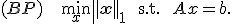(1)

Basis pursuit denoise(Three forms).

BP is relaxed to obtain the basis pursuit denoise (BPDN) problem.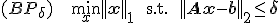(2)
The parameteris used to estimate the noise level of the input data sets.It becomes a standard BP problem whenequals to  zero.
E.V.D. Berg, etl, have proposed an efficient algorithm for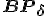problem.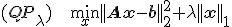(3)
Using Lagrange-operater into problem (2), it turn problem (2) into problem (3).
It is first proposed by Chen, Donoho, and Sunders.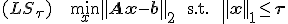It was proposed by R. T.IBSHIRNI.

For the case where an estimate of the noise levelis known, Chen, Donoho, etl  argue that the choice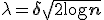has important optimality properties.

Reference

  D. L. Donoho, For most large underdetermined systems of linear equations the minimal 1-
norm solution is also the sparsest solution,        Comm. Pure Appl. Math., 59 (2006), pp. 797–829.
   S. S. Chen, D. L. Donoho, and M. A. Saunders, Atomic decomposition by basis pursuit,SIAM Rev.   43 (2001), pp. 129–159.
   E.V.D. Berg and M.P. Friedlander,  Probing the pareto frontier for basis puisuit solutions.  SIAM J. Sci. Comput.     31(2008), pp.  890-912.
     R. Tibshirani, Regression shrinkage and selection via the Lasso, J. Roy. Statist. Soc. Ser. B.    58 (1996), pp. 267–288.

http://blog.sciencenet.cn/blog-621576-551341.html

## 相关博文

GMT+8, 2021-4-13 03:37

Powered by ScienceNet.cn

Copyright © 2007- 中国科学报社